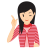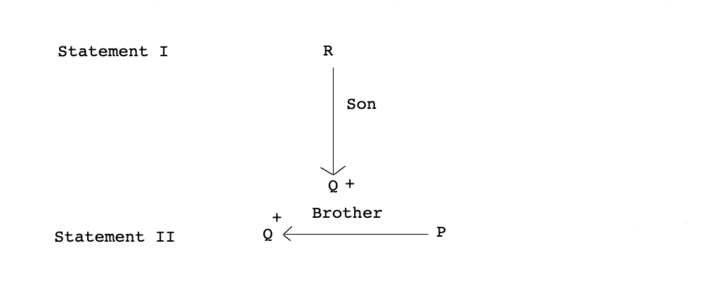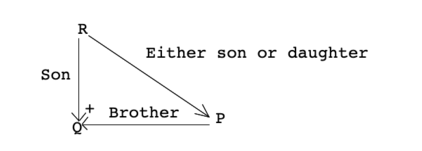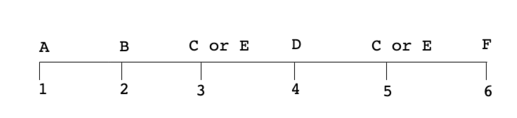# Data Sufficiency

Data Sufficiency is a method to analyse the given set of information and decide whether the given information is sufficiency to answer the question. Data sufficiency questions include problems based on Coding-Decoding, Blood Relations, Direction Sense Test, Ranking and Time Sequence Test, Arithmetical Reasoning, Mathematical Operations and Puzzle Test etc.Few Important things to Remember

The problems based on this topic consist of two or more than two statements containing the information related to it. You have to decide whether the problem can be solved by using the information from the given statements combined or individually.

In these questions, it is also possible that either of the given statements is sufficient to answer the question.

Directions (Example Nos. 1-4)The questions given below consist of a question followed by two statements labelled as I and II. We have to decide whether these statements given enough information required to answer the question or not.

(a) If Statement I alone is sufficient to answer the question

(b) If Statement II alone is sufficient to answer the question

(c) If Statements I and II together are needed to answer the question

(d) If statement I or II alone is sufficient to answer the question

(e) If Statements I and II together are not sufficient to answer the question

Example - 1 How is P related to R?

I Q is the son of R.

II Q is the brother of P.

Solution: (e)Now, from both statements,Here, P is either the son or daughter of R. So, we cannot find the exact relation between P and R.

Hence, Statement I and II together are not sufficient to answer the question.

Example - 2  Who is older Ritu or Situ?

I.  Situ was born in the year 1980 and is 5yr younger than Ritu's brother.

II. Ritu is twice as old as her brother.

Solution: (c)  From Statement I, it is clear that Situ is younger than Ritu's brother.

From Statement II, it is clear that Ritu is older than her brother.

So, from both the statements, we get

Ritu > Ritu's Brother > Situ

Hence, Ritu is older than Situ.

Example - 3  What is the sum of first two even natural numbers out of a group of five even natural numbers?

I. The second number is double of the first number.

II. All the five numbers are consecutive even numbers.

Solution: (c)  From Statement II, the five numbers are consecutive even numbers.

From Statement I, second number is double of first number. On combining both Statement I and II, we get

First number = 2 and second number = 4

[since, this is the only possibility in case of consecutive even numbers, where second number is double of the first number]Sum of first two numbers = 2 + 4 = 6

So, both Statements I and II are required to answer the question.

Example - 4  Six persons A, B, C, D, E and F are sitting in a row facing North. A and F are sitting at two extreme ends of the row. B is to the immediate right of A and D is 2nd left of F.
What is the position of E with respect to A?

I. E is to the right of A.

II. E is to the left of C.

Solution: (b)  On the basis of the statements given in the question, we can arrange the person in a row in the following way

Positions of C and E can be either 3rd or 5th.From the Statement I, we get that E is to the right of A and hence, position of E can either be 3rd or 5th.

Therefore, Statement I alone is not sufficient to answer the question. Statement II fixed the position of C at 5th and E at 3rd place, respectively. Therefore, we get our answer from Statement II.

Directions (Example Nos. 5) In the question given below, three Statements I, II and III are given. You are required to find out which of the given statements is / are sufficient to answer the question.

Example - 5  On which day Suresh went to Chennai, if week starts on Monday?

(a) II and III

(b) I and II

(c) I and III

(d) All are needed

(e) Even I, II and III together are not sufficient

Solution: (e)  Statements I, II and III together are not sufficient as the required day can be anyone of Wednesday, Thursday, Friday and Sunday.

LearnFrenzy provides you lots of fully solved Data Sufficiency Questions and Answers with explanation.